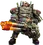Area Damage is, as its name implies, any type of damage being dealt in an area. The damage done in that area may not be the same everywhere. For most weapons, more damage will be done near the center than at the edges. The damage done isn’t affected by what is inside that area and will remain the same no matter the number of enemies.

## Common sources

The most common source of Area Damage in game comes in the form of explosive damage, but in practice any attack that deals damage in a sphere will behave the same.
Examples of non explosive Area Damage include the second part of the melee attack from the Pickaxe (Melee damage), the active See You In Hell (Disintegrate damage), Death Cloud explosion (Fire + Heat damage).
Area Damage can also come from attacks that don't do any health damage but affect temperature instead like the Cryo Cannon's Cold Radiance mod and Snowball overclock.

## Terminology• Area Damage: This is the maximum amount of damage that will be done, usually in a small radius at the center of the area (in red).
• Maximum Damage Radius: Read this as Radius where the Maximum Damage will be done. This is a, usually smaller, part of the area where the damage done will be equal to the Area Damage (in red).
• Radius: A concentric radius, usually bigger than the Maximum Damage Radius. At the edge of this area, you will do the minimum possible damage (in yellow).
• Minimum Area Damage: The damage done at the edge of the Radius is determined as a percentage of the Area Damage. By default that value is 25%, but most weapons use a custom value (in blue).

The damage will scale linearly between the two radii (in yellow).

## Mods and Overclock Increasing Radius

Any Mod or Overclock that increase the Radius in game will only affect the Radius, not the Maximum Damage Radius.
Only the Area Damage and Radius stats are visible in game; the Maximum Damage Radius and Minimum Area Damage are hidden.

Deepcore 40mm PGL
Without mods With Nails + Tape (Radius +1m)Area Damage: 110 Explosive damage

Minimum Area Damage: 50 %Area Damage: 110 Explosive damage

Minimum Area Damage: 50 %

## Calculating the Damage Done at Any Range

We will take as an example a weapon with the following stats:Area Damage: 60 Explosive damage
Minimum Area Damage: 20 %

That means that weapon will deal:

• 60 Explosive damage within a sphere of 2m or less (in the red area).
• 20% of 60 = 12 Explosive damage at exactly 4m (on the blue line).
• No damage beyond 4m (beyond the blue line).
• Damage scales linearly between 2m and 4m (in the yellow area).

To calculate the damage done, since we know the damage scales between the two radii, that mean that the ratio of area damagewill be equal to the corresponding ratio of radius.

From that point we can can deduce a formula to calculate the damage done Damage(x) depending on the distance from the center of the area x:Using the above formula, we can find now find the damage done at any point inside the scaling area:## Special Case

If for any reason the Radius is smaller than the Maximum Damage Radius (e.g. Deepcore 40mm PGL with the Overclock Hyper Propellant), then full damage will be done inside the Radius and no damage will be done between the Radius and Maximum Damage Radius.